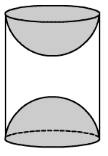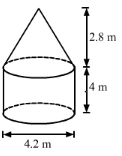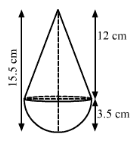RS Aggarwal Class 10 Solutions Chapter 19 - Volume And Surface Areas Of Solids Ex 19 G (19.7)

RS Aggarwal Class 10 Chapter 19 - Volume And Surface Areas Of Solids Ex 19 G (19.7) Solutions Free PDF

Question 1: A copper sphere of diameter 18 cm is drawn into wire of diameter 4 mm. Find the length of the wire.

Solution:

We have, Radius of the sphere, R = $\frac{18}{2}$ = 9 cm and

Radius of the wire, r = $\frac{4}{2}$ = 2 mm = 0.2 cm

Let the length of the wire be l.

Now,

Volume of the wire = Volume of the copper sphere

$\Rightarrow$ $\Pi r^{2}l=\frac{4}{3}\Pi R^{3}$

$\Rightarrow$ l = $\frac{4R^{3}}{3r^{2}}$

$\Rightarrow$ l = $\frac{4\times 9\times 9\times 9}{3\times 0.2\times 0.2}$

Therefore, l = 24300 cm = 243 m

So, the length of the wire is 243 m.

Question 2: The radii of the circular ends of a frustum of height 6 cm are 14 cm and 6 cm respectively. Find the slant height of the frustum.

Solution:

We have,

Height of the frustum, h = 6 cm,

Radii of the circular ends, R = 14 cm and r = 6 cm

Let the slant height of the frustum be l.

Now,

l = $\sqrt{(R-r)^{2}+h^{2}}$

= $\sqrt{(14-6)^{2}+6^{2}}$

= $\sqrt{8^{2}+6^{2}}$

= $\sqrt{64+36}$

= $\sqrt{100}$

= 10 cm

So, the slant height of the frustum is 10 cm.

Question 3: Find the ratio of the volume of a cube to that of a sphere which will fit inside it.

Solution:

Let the radius of the sphere be R and the edge of the cube be a.

As, the sphere is fit inside the cube.

So, diameter of the sphere = edge of the cube

$\Rightarrow$ 2R = a – – – – – – – – – – – (i)

Now,

The ratio of the volume of the cube to that of the sphere = $\frac{Volume\, of\, the\, cube}{Volume\, of\, the\, sphere}$

= $\frac{a^{3}}{\left ( \frac{4}{3}\Pi R^{3} \right )}$

= $\frac{(2R)^{3}}{\left ( \frac{4}{3}\Pi R^{3} \right )}$ [Using (i)]

= $\frac{3\times 8R^{3}}{4\Pi R^{3}}$

= $\frac{6}{\Pi }$

= 6 : $\Pi$

So, the ratio of the volume of the cube to that of the sphere is 6 : $\Pi$

Question 4: Find the ratio of the volumes of a cylinder, a cone and a sphere, if each has the same diameter and same height?

Solution:

Let the radius of the sphere be r.

We have,

The radius of the cone = The radius of the cylinder = The radius of the sphere = r

and

The height of the cylinder = The height of the cone = The height of the sphere = 2r

Now,

Volume of the cylinder = $\Pi r^{2}(2r)=2\Pi r^{3}$,

Volume of the cone = $\frac{1}{3}\Pi r^{2}(2r)=\frac{2}{3}\Pi r^{3}$ and

Volume of the sphere = $\frac{4}{3}\Pi r^{3}$

So,

The ratio of the volumes of the cylinder, the cone and the sphere = $2\Pi r^{3}:\frac{2}{3}\Pi r^{3}:\frac{4}{3}\Pi r^{3}$

= $1:\frac{1}{3}:\frac{2}{3}$

= 3 : 1 : 2

So, the ratio of the volumes of the cylinder, the cone and the sphere is 3 : 1 : 2.

Question 5: Two cubes each of volume 125 cm3 are joined end to end to form a solid. Find the surface area of the resulting cuboid.

Solution:

Let the edge of the cube be a.

As, Volume of the cube = 125 cm3

$\Rightarrow$ a3 = 125

$\Rightarrow$ a = $\sqrt{125}$

$\Rightarrow$ a = 5 cm

So,

Length of the resulting cuboid, l = 2 x 5 = 10 cm,

Breadth of the resulting cuboid, b = 5 cm and

Height of the resulting cuboid, h = 5 cm

Now,

Surface area of the resulting cuboid = 2(lb + bh + hl)

= 2 x (10 x 5 + 5 x 5 + 5 x 10)

= 2 x (50 + 25 + 50)

= 2 x 125

= 250 cm2

So, the surface area of the resulting cuboid is 250 cm2.

Question 6: Three metallic cubes whose edges are 3 cm, 4 cm and 5 cm, are melted and recast into a single large cube. Find the edge of the new cube formed.

Solution:

We have,

Edges of the cubes : a1 = 3 cm, a2 = 4 cm and a3 = 5 cm

Let the edge of the new cube be a.

Now,

Volume of the new cube = a13 + a23 + a33

$\Rightarrow$ a3 = 33 + 43 + 53

$\Rightarrow$ a3 = 27 + 64 + 125

$\Rightarrow$ a3 = 216

$\Rightarrow$ a = $\sqrt{216}$

Therefore, a = 6 cm

So, the edge of the new cube so formed is 6 cm.

Question 7: A solid metallic sphere of diameter 8 cm is melted and drawn into a cylindrical wire of uniform width. If the length of the wire is 12 m, find its width.

Solution:

We have,

Radius of the metallic sphere, R = $\frac{8}{2}$ = 4 cm and

Height of the cylindrical wire, h = 12 m = 1200 cm

Let the radius of the base be r.

Now,

Volume of the cylindrical wire = Volume of the metallic sphere

$\Rightarrow$ $\Pi r^{2}h=\frac{4}{3}\Pi R^{3}$

$\Rightarrow$ r2 = $\frac{4R^{3}}{3h}$

$\Rightarrow$ r2 = $\frac{4\times 4\times 4\times 4}{3\times 1200}$

$\Rightarrow$ r2 = $\frac{16}{225}$

$\Rightarrow$ r = $\sqrt{\frac{16}{225}}$

$\Rightarrow$ r = $\frac{4}{15}cm$

Therefore, The width of the wire = 2r

= $2\times \frac{4}{15}$

= $\frac{8}{15}cm$

So, the width of the wire is $\frac{8}{15}$ cm.

Question 8: A 5-m-wide cloth is used to make a conical tent of base diameter 14 m and height 24 m. Find the cost of cloth used, at the rate of Rs. 25 per meter.

Solution:

We have,

Width of the cloth, B = 5 m,

Radius of the conical tent, r = $\frac{14}{2}$ = 7 m and

Height of the conical tent, h = 24 m

Let the length of the cloth used for making the tent be L.

Also,

The slant height of the conical tent, l = $\sqrt{r^{2}+h^{2}}$

= $\sqrt{7^{2}+24^{2}}$

= $\sqrt{49+576}$

= $\sqrt{625}$

= 25 m

Now,

The curved surface of the conical tent = $\Pi rl$

= $\frac{22}{7}\times 7\times 25$

$\Rightarrow$ The area of the cloth used for making the tent = 550 m2

$\Rightarrow$ LB = 550

$\Rightarrow$ L = $\frac{550}{B}$

$\Rightarrow$ L = $\frac{550}{5}$

$\Rightarrow$ L = 110 m

So, the cost of the cloth used = 25 x 110 = 2750

So, the cost of the cloth used for making the tent is Rs. 2750.

Question 9: A wooden toy was made by scooping out a hemisphere of the same radius from each end of a solid cylinder. If the height of the cylinder is 10 cm and its base is of radius 3.5 cm, find the volume of wood in the toy.

Solution:We have,

Radius of the cylinder = Radius of the hemisphere = r = 3.5 cm and

Height of the cylinder, h = 10 cm

Now,

Volume of the toy = Volume of the cylinder – Volume of the two hemispheres

= $\Pi r^{2}h-2\times \frac{2}{3}\Pi r^{3}$

= $\Pi r^{2}\left ( h-\frac{4r}{3} \right )$

= $\frac{22}{7}\times 3.5\times 3.5\times \left ( 10-\frac{4\times 3.5}{3} \right )$

= $38.5\times \left ( 10-\frac{14}{3} \right )$

= $38.5\times \frac{16}{3}$

= $\frac{616}{3}cm^{3}$

$\approx 205.33cm^{3}$

So, the volume of wood in the toy is $\frac{616}{3}cm^{3}$ or 205.33 cm3.

Question 10: Three cubes of a metal whose edges are in the ratio 3 : 4 : 5 are melted and converted into a single cube whose diagonal is $12\sqrt{3}$ cm. Find the edges of the three cubes.

Solution:

Let the edge of the metal cubes be 3x, 4x and 5x.

Let the edge of the single cube be a.

As,

Diagonal of the single cube = $12\sqrt{3}$ cm

$\Rightarrow$ $a\sqrt{3}=12\sqrt{3}$

$\Rightarrow$ a = 12 cm

Now,

Volume of the single cube = Sum of the volumes of the metallic cubes

$\Rightarrow$ a3 = (3x)3 + (4x)3 + (5x)3

$\Rightarrow$ 123 = 27x3 + 64x3 + 125x3

$\Rightarrow$ 1738 = 216x3

$\Rightarrow$ x3 = $\frac{1728}{216}$

$\Rightarrow$ x3 = 8

$\Rightarrow$ x = $\sqrt{8}$

$\Rightarrow$ x = 2

So, the edges of the cubes are 3 x 2 = 6 cm, 4 x 2 = 8 cm and 5 x 2 = 10 cm.

Hence, the edges of the given three metallic cubes are 6 cm, 8 cm and 10 cm.

Question 11: A hollow sphere of external and internal diameters 8 cm and 4 cm respectively is melted into a solid cone of base diameter 8 cm. Find the height of the cone.

Solution:

We have,

External radius of the hollow sphere, R1 = $\frac{8}{2}$ = 4 cm,

Internal radius of the hollow sphere, R2 = $\frac{4}{2}$ = 2 cm and

Base radius of the cone, r = $\frac{8}{2}$ = 4 cm

Let the height of the cone be h.

Now,

Volume of the cone = Volume of the hollow sphere

$\Rightarrow$ $\frac{1}{3}\Pi r^{2}h=\frac{4}{3}\Pi R_{1}^{3}-\frac{4}{3}\Pi R_{2}^{3}$

$\Rightarrow$ $\frac{1}{3}\Pi r^{2}h=\frac{4}{3}\Pi \left ( R_{1}^{3}- R_{2}^{3}\right )$

$\Rightarrow$ h = $\frac{4}{r^{2}}\left ( R_{1}^{3}- R_{2}^{3} \right )$

$\Rightarrow$ h = $\frac{4}{4\times 4}\left ( 4^{3}- 2^{3} \right )$

$\Rightarrow$ h = $\frac{1}{4}\left ( 64-8 \right )$

$\Rightarrow$ h = $\frac{1}{4}\times 56$

Therefore, h = 14 cm

So, the height of the cone is 14 cm.

Question 12: A bucket of height 24 cm is in the form of the frustum of a cone whose circular ends are of diameter 28 cm and 42 cm. Find the cost of milk at the rate of Rs. 30 per liter, which the bucket can hold.

Solution:

We have,

Height of the frustum, h = 24 cm,

Radius of the open end, R = $\frac{42}{2}$ = 21 cm and

Radius of the close end, r = $\frac{28}{2}$ = 14 cm

Now,

Volume of the bucket = $\frac{1}{3}\Pi h(R^{2}+r^{2}+Rr)$

= $\frac{1}{3}\times \frac{22}{7}\times 24\times (21^{2}+12^{2}+21\times 14)$

= $\frac{176}{7}\times (441+196+294)$

= $\frac{176}{7}\times 931$

= 23408 cm3

= 23.408 L (As, 1000 cm3 = 1 L)

Therefore, The cost of the milk = 30 x 23.408 = 702.24

So, the cost of the milk which the bucket can hold is Rs. 702.24.

Question 13: The interior of a building is in the form of a right circular cylinder of diameter 4.2 m and height 4 m surmounted by a cone of the same diameter. The height of the cone is 2.8 m. Find the outer surface area of the building.

Solution:We have,

Radius of the cylinder = Radius of the cone = r = $\frac{4.2}{2}$ = 2.1 m,

Height of the cylinder, H = 4 m and

Height of the cone, h = 2.8 m

Also,

The slant height of the cone, l = $\sqrt{r^{2}+h^{2}}$

= $\sqrt{2.1^{2}+2.8^{2}}$

= $\sqrt{4.41+7.84}$

= $\sqrt{12.25}$

= 3.5 m

Now,

The outer surface area of the building = CSA of the cylinder + CSA of the cone

= $2\Pi rH+\Pi rl$

= $\Pi r(2H+l)$

= $\frac{22}{7}\times 2.1\times (2\times 4+3.5)$

= 6.6 x 11.5

= 75.9 m2

So, the outer surface area of the building is 75.9 m2.

Question 14: A metallic solid right circular cone is of height 84 cm and the radius of its base is 21 cm. It is melted and recast into a solid sphere. Find the diameter of the sphere.

Solution:

We have,

Height of the cone, h = 84 cm and

Base radius of the cone, r = 21 cm

Let the radius of the solid sphere be R.

Now,

Volume of the solid sphere = Volume of the solid cone

$\Rightarrow$ $\frac{4}{3}\Pi R^{3}=\frac{1}{3}\Pi r^{2}h$

$\Rightarrow$ R3 = $\frac{r^{2}h}{4}$

$\Rightarrow$ R3 = $\frac{21\times 21\times 84}{4}$

$\Rightarrow$ R3 = 21 x 21 x 21

$\Rightarrow$ R = 21 cm

Therefore, Diameter = 2R = 2 x 21 = 42 cm

So, the diameter of the solid sphere is 42 cm.

Question 15: A toy is in the form of a cone of radius 3.5 cm mounted on a hemisphere of the same radius. The total height of the toy is 15.5 cm. Find the total surface area of the toy.

Solution:We have,

Radius of the hemisphere = Radius of the cone = r = 3.5 cm and

Height of the cone = 15.5 – 3.5 = 12 cm

Also,

The slant height of the cone, l = $\sqrt{h^{2}+r^{2}}$

= $\sqrt{12^{2}+3.5^{2}}$

= $\sqrt{144+12.25}$

= $\sqrt{156.25}$

= 12.5 cm

Now,

Total surface area of the toy = CSA of cone + CSA of hemisphere

= $\Pi rl+2\Pi r^{2}$

= $\Pi r(l+2r)$

= $\frac{22}{7}\times 3.5\times (12.5+2\times 3.5)$

= 11 x (12.5 + 7)

= 11 x 19.5

= 214.5 cm2

So, the total surface area of the toy is 214.5 cm2.

Question 16: If the radii of the circular ends of a bucket 28 cm high, are 28 cm and 7 cm, find its capacity and total surface area.

Solution:

We have,

Height, h = 28 cm,

Radius of the upper end, R = 28 cm and

Radius of the lower end, r = 7 cm

Also,

The slant height, l = $\sqrt{(R-r)^{2}+h^{2}}$

= $\sqrt{(28-7)^{2}+28^{2}}$

= $\sqrt{21^{2}+28^{2}}$

= $\sqrt{441+784}$

= $\sqrt{1225}$

= 35 cm

Now,

Capacity of the bucket = $\frac{1}{3}\Pi h(R^{2}+r^{2}+Rr)$

= $\frac{1}{3}\times \frac{22}{7}\times 28\times (28^{2}+7^{2}+28\times 7)$

= $\frac{88}{3}\times (784+49+196)$

= $\frac{88}{3}\times 1029$

= 30184 cm3

Also,

Total surface area of the bucket = $\Pi l(R+r)+\Pi r^{2}$

= $\frac{22}{7}\times 35\times (28+7)+\frac{22}{7}\times 7\times 7$

= 110 x (35) + 154

= 4004 cm2

Question 17: A bucket is in the form of a frustum of a cone with a capacity of 12308.8 cm3 of water. The radii of the top and bottom circular ends are 20 cm and 12 cm respectively. Find the height of the bucket. (Use $\Pi =3.14$)

Solution:

We have,

Radius of the upper end, R = 20 cm and

Radius of the lower end, r = 12 cm

Let the height of the bucket be h.

As,

Volume of the bucket = 12308.8 cm3

$\Rightarrow$ $\frac{1}{3}\Pi h(R^{2}+r^{2}+Rr)$ = 12308.8

$\Rightarrow$ $\frac{1}{3}\times 3.14\times h\times (20^{2}+12^{2}+20\times 12)$ = 12308.8

$\Rightarrow$ $\frac{3.14h}{3}\times (400+144+240)=12308.8$

$\Rightarrow$ $\frac{3.14h}{3}\times 784=12308.8$

$\Rightarrow$ h = $\frac{12308.8\times 3}{3.14\times 784}$

Therefore, h = 15 cm

So, the height of the bucket is 15 cm.

Question 18: A milk container is made of metal sheet in the shape of the frustum of a cone whose volume is $10459\frac{3}{7}$ cm3. The radii of its lower and upper circular ends are 8 cm and 20 cm respectively. Find the cost of metal sheet used in making the container at the rate of Rs. 1.40 cm2.

Solution:

We have,

Radius of the upper end, R = 20 cm and

Radius of the lower end, r = 8 cm

Let the height of the container be h.

As,

Volume of the container = $10459\frac{3}{7}$ cm3

$\Rightarrow$ $\frac{1}{3}\Pi h(R^{2}+r^{2}+Rr)$ = $\frac{73216}{7}$

$\Rightarrow$ $\frac{1}{3}\times \frac{22}{7}\times h\times (20^{2}+8^{2}+20\times 8)$ = $\frac{73216}{7}$

$\Rightarrow$ $\frac{22h}{21}\times (400+64+160)$ = $\frac{73216}{7}$

$\Rightarrow$ $\frac{22h}{21}\times 624$ = $\frac{73216}{7}$

$\Rightarrow$ h = $\frac{73216\times 21}{7\times 22\times 624}$

$\Rightarrow$ h = 16 cm

Also,

The slant height of the container, l = $\sqrt{(R-r)^{2}+h^{2}}$

= $\sqrt{(20-8)^{2}+16^{2}}$

= $\sqrt{12^{2}+16^{2}}$

= $\sqrt{144+256}$

= $\sqrt{400}$

= 20 cm

Now,

Total surface area of the container = $\Pi l(R+r)+\Pi r^{2}$

= $\frac{22}{7}\times 20\times (20+8)+\frac{22}{7}\times 8\times 8$

= $\frac{22}{7}\times 20\times 28+\frac{22}{7}\times 64$

= $\frac{22}{7}\times (560+64)$

= $\frac{22}{7}\times 624$

= $\frac{13728}{7}$ cm2

So, the cost of metal sheet = $1.4\times \frac{13728}{7}=2745.60$

Hence, the cost of the metal sheet used for making the milk container is Rs. 2745.60.

Question 19: A solid metallic sphere of diameter 28 cm is melted and recast into a number of smaller cones, each of diameter $4\frac{2}{3}$ and height 3 cm. Find the number of cones so formed.

Solution:

We have,

Radius of the metallic sphere, R = $\frac{28}{2}$ = 14 cm,

Radius of the smaller cone, r = $\frac{1}{2}\times \left ( 4\frac{2}{3} \right )=\frac{1}{2}\times \frac{14}{3}=\frac{7}{3}$ cm and

Height of the smaller cone, h = 3 cm

Now,

The number of cones so formed = $\frac{Volume\, of\, the\, metallic\, sphere}{Volume\, of\, a\, smaller\, cone}$

= $\frac{\left ( \frac{4}{3}\Pi R^{3} \right )}{\left ( \frac{1}{3}\Pi r^{2}h \right )}$

= $\frac{4R^{3}}{r^{2}h}$

= $\frac{4\times 14\times 14\times 14}{\left ( \frac{7}{3}\times \frac{7}{3}\times 3 \right )}$ = 672

So, the number of cones so formed is 672.

Question 20: A cylindrical vessel with internal diameter 10 cm and height 10.5 cm is full of water. A solid cone of base diameter 7 cm and height 6 cm is completely immersed in water. Find the volume of water

(i) displaced out of the cylinder

(ii) left in the cylinder.

Solution:

We have,

Internal radius of the cylindrical vessel, R = $\frac{10}{2}$ = 5 cm,

Height of the cylindrical vessel, H = 10.5 cm,

Radius of the solid cone, r = $\frac{7}{2}$ = 3.5 cm and

Height of the solid cone, h = 6 cm

(i) Volume of water displaced out of the cylinder = Volume of the solid cone

= $\frac{1}{3}\Pi r^{2}h$

= $\frac{1}{3}\times \frac{22}{7}\times 3.5\times 3.5\times 6$ = 77 cm3

(ii) As, Volume of the cylindrical vessel = $\Pi R^{2}H$

= $\frac{22}{7}\times 5\times 5\times 10.5$

= 825 cm3

So, the volume of water left in the cylindrical vessel = Volume of the cylindrical vessel – Volume of the solid cone

= 825 – 77 = 748 cm3

Practise This Question

What is the nature of image formed on the retina of the eye?